# Free SAT II Physics Practice Solutions on DC Electric Circuits

 Solutions to the Sat Physics subject questions on on DC electric circuits, with detailed explanations. The circuit below is related to questions 1 and 2. V is the e.m.f of the battery, 2R and R are resistors such that the resistance of 2R is double the resistance of R.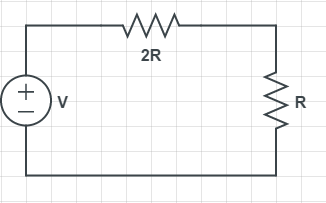Fig1. - Electric Circuit Questions 1 and 2. The voltage across resistor R above is given by A) 3V B) V/3 C) V D) V/4 E) V/2 Solution - Explanations current in the above circuit = I = V / (2 R + R) = V / (3 R) Voltage across R = U = R I = R V / (3 R) = V / 3 Answer B. If W is the amount of heat generated during 10 seconds in resistor R included in the circuit in figure 1 above, what would be the amount of heat generated across the same resistor during 20 seconds if V is doubled? A) W B) 2W C) 4W D) 8W E) 80W Solution - Explanations W = time � power = 10 U I = 10 ( V / 3) (V / (3 R)) (see I and U calculated in questions 1 above) W = 10 V2 / (9 R) (after simplification) if V is doubled to 2 V, during 20 seconds, the energy W2 is given by W2 = 20 (2 V) 2 / (9 R) = 80 V2 / (9R) = 8 W Answer D. What is the equivalent resistance between points A and B in the circuit below?A) 0.5 R B) R C) 2 R D) 3 R E) 4 R Solution - Explanations Let Re be the equivalent resistance to the two parallel 2R resistors. 1/Re = 1 / 2R + 1 / 2R = 2 / (2 R) = 1 / RHence Re = R The equivalent resistance RAB between points A and B is given by RAB = R + Re = R + R = 2 R ( R and Re are in series) Answer C. Find resistance x, in the circuit below, so that the power generated across resistor x is 80% of the total power across resistors R and x?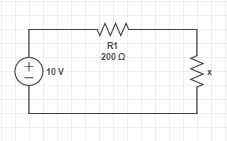A) 100 Ω B) 200 Ω C) 400 Ω D) 600 Ω E) 800 Ω Solution - Explanations Let I be the current across R1 and x. Let P1 be the power across x and P2 the power across R1 and x. P1 = x I2 , P2 = (x + 200) I 2 P1 = 80% P2 gives x I2 = 80% (x + 200) I 2 Simplify to obtain x = 0.8 (x + 200) Solve for x to obtain x = 800 Ω Answer E. Two resistors R1 and R2 such that R2 = 3 R1 are in parallel. What is the ratio of the power generated across R1 to the power generated across R2? A) 1 B) 9 C) 3 D) 1 / 3 E) 1 / 9 Solution - Explanations Let P1 be the power across R1 and P2 the power across R2. Since R1 and R2 are in parallel, the voltage U across both resistors is the same. Hence: P1 = U2 / R1 and P2 = U2 / R2 = U2 / (3 R1) P1 / P2 = [ U2 / R1 ] / [ U2 / (3 R1) ] Simplify to obtain : P1 / P2 = 3 Answer C. What is the equivalent capacitance between points A and B?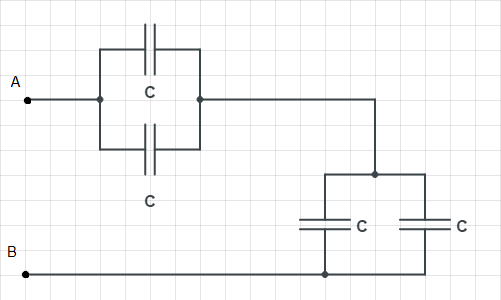A) C / 2 B) C C) 2 C D) 3 C E) 4 C Solution - Explanations The upper two capacitors are in parallel and their equivalent capacitance is 2 C The two capacitors on the right are also in parallel and their equivalent capacitance is 2 C (see circuit below)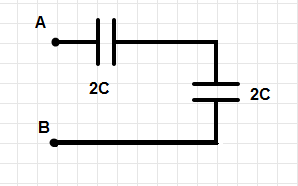We now have the two capacitors each of capacitance 2 C in series and the equivalent capacitance Ce is given by 1 / Ce = 1 / 2C + 1 / 2C = 2 / 2C = 1 / C Ce = C Answer B If 20 Joules of heat is produced across a resistor R during 50 seconds, what amount of heat is produced across the same resistance during 100 seconds if the current across it is doubled? A) 10 J B) 20 J C) 40 J D) 80 J E) 160 J Solution - Explanations Let I be the current across R and W the heat generated during 50 seconds. Hence W = 50 R I2 = 20 J If I is doubled, the energy W2 generated during 100 seconds is given by W2 = 100 (2 I)2 R = 400 I2 R = 8(50 R I2) = 8 * 20 J = 160 J Answer E. Which of the following is true about electric energy? A) It is stored in resistors. B) It cannot be stored at all. C) It is stored in capacitors. D) It is stored in conducting wires. E) None of the above. Solution - Explanations Electric energy may be stored in capacitors Answer C. Once the switch S1 is closed in the circuit below, which of the following is true about the current through the resistor R1?A) It increases from 0 to V / R1. B) It decreases from V / R1 to 0. C) It stays constant and equal to V / R1. D) It stays constant and equal to (1/2)( V / R1). E) It stays constant and equal to 0. Solution - Explanations As soon as the switch is closed (t=0), current through the resistor R1 is given by V / R1. Then as time increases, the capacitor charge increases, the current through R start decreasing exponentially to 0. Answer B. Which of the following is true about the circuit below if R2 > R3?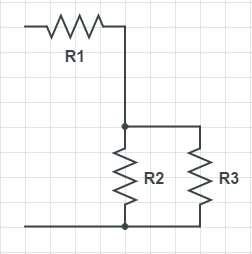I) The current across R1 is larger than the current across R2 II) The voltage across R3 is equal to the voltage across R2 III) The current across R2 is larger than the current across R3 A) I) and II) only B) II) only C) III) only D) I) only E) I) II) and III) Solution - Explanations I) is true: the current through R1 is the sum of the currents through R2 and R3. Hence the current through R1 is larger than the current in R2 and the current in R3. II) is true, voltage is measured between two points which in this case are common to the two resistors. III) not true: Let U be the voltage across R2 and R3. The current across R2 is given by U / R2 and the current across R3 is given by U / R3. Since R2 > R3, U / R2 is smaller than U / R3. Answer A. Answers to the Above questions B D C E C B E C B A
Share
Popular Pages